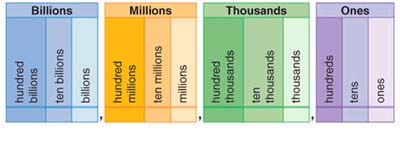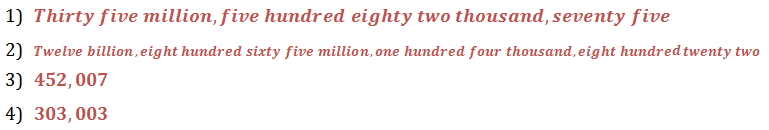Lesson - Place Value

Place Value

When a whole number is written using the digits 0, 1, 2, 3, 4, 5, 6, 7, 8, and 9, it is said to be in standard form. The position of each digit determines the digit's place value . A place value chart names each place value.

When a number is written in standard form, each group of digits separated by a comma is called a period . The number 5,913,603,800 has four periods. Each period is shown by a different color in the place value chart. The period name is written above each period.To write a whole number in standard form, write the number named in each period, and replace each period name with a comma.

5,913,603,800 is read "five billion, nine hundred thirteen million, six hundred three thousand, eight hundred."

Practice

1. Write 35,582,075 in words.

2. Write 12,865,104,822 in words.

3. Write four hundred fifty-two thousand seven in standard form.

4. Write three hundred three thousand three in standard form.